# Problems on similar triangles for class 10

Given below are the Similar Triangles Class 10 Problems and questions with Answer
a. Proof Questions
b. Calculation problems
c. True & False Questions
d. Fill in the blanks

## Proof Problems/word Problems

Question 1.
In $\Delta ABC$ , ray AD bisects $\angle A$ and intersects BC in D. If BC = a, AC = b and AB = c, prove that:
i. $BD = \frac {ac}{b+c}$
ii.$DC = \frac {ab}{c+b}$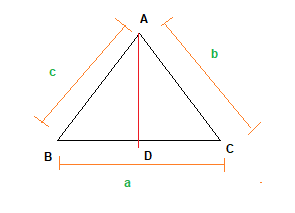From Angle Bisector theorem
$\frac {AC}{AB} = \frac {CD}{BD}$
$\frac {b}{c}=\frac {CD}{BD}$
$\frac {b}{c} + 1 =\frac {CD}{BD} +1$
$\frac {b+c}{c}= \frac {CD+BD}{BD}$
$\frac {b+c}{c}= \frac {BC}{BD}$
$\frac {b+c}{c}= \frac {a}{BD}$
$BD= \frac {ac}{b+c}$

Now,
$CD = BC -BD$
$CD= a - \frac {ac}{b+c}$
$DC = \frac {ab}{b+c}$

Question 2.
Two poles of heights a and b metres are standing vertically on a level ground r metres apart. Prove that the height c of the point of intersection of the lines joining the top of each pole to the foot of the opposite pole is given by $\frac {ab}{a+b}$ i.e $c = \frac {ab}{a+b}$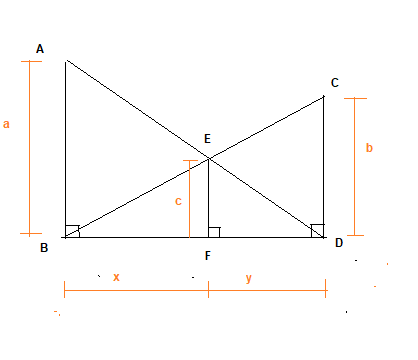Let FB=x and FD=y
Now in $\Delta ABD$ and $\Delta EFD$
$\angle ABD= \angle EFD$
$\angle ADB= \angle EDF$ (common angle)
By SS criteria,
$\Delta ABD \sim \Delta EFD$
$\frac {AB}{EF} = \frac {BD}{FD}$
$\frac {a}{c} = \frac {x+y}{y}$
$\frac {a}{c} -1 = \frac {x+y}{y} -1$
$\frac {a-c}{c}= \frac {x}{y}$ -(1)

Now in $\Delta CDB$ and $\Delta EFB$

$\angle CDB= \angle EFB$
$\angle CBD= \angle EBF$ (common angle)
By SS criterio,
$\Delta CDB \sim \Delta EFB$
$\frac {CD}{EF} = \frac {BD}{FB}$
$\frac {b}{c} = \frac {x+y}{x}$
$\frac {b-c}{c} = \frac {y}{x}$
or $\frac {x}{y} = \frac {c}{b-c}$ -(2)
From (1) and (2)
$\frac {a-c}{c}=\frac {c}{b-c}$
$(a-c)(b-c)=c^2$
$ab -ac -bc + c^2=c^2$
$c= \frac {ab}{a+b}$

Question 3.
D and E are points on the sides CA and CB respectively of $\Delta ABC$ right-angled at C. Prove that $AE^2 + BD^2 = AB^2 + DE^2$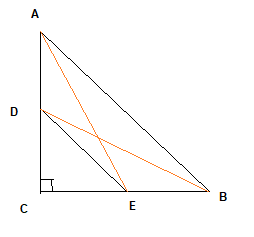This can be easily solved using Pythagorus theorem
In Right angle triangle ACE,By Pythagoras Theorem
$AC^2+ CE^2�= AE^2$� --(1) � �
In Right angle triangle DBC,By Pythagoras Theorem
$DC^2+ BC^2 =�BD^2$�-(2) �
In Right angle triangle ABC,By Pythagoras Theorem
$AC^2�+ BC^2�= AB^2$ ---(3) ���

In Right angle triangle DEC,By Pythagoras Theorem
$DC^2�+ CE^2�= DE^2$�---(4) �

? Adding (1) and (2) we get,
$AE^2�+ BD^2�= AC^2�+ CE^2+ DC^2+ BC^2$
$= (AC^2�+ BC^2) + (CE^2+ DC^2)$

Now from (3) and (4)
$AE^2 + BD^2 = AB^2 + DE^2$

Question 4.
In a quadrilateral ABCD, $\angle B = 90$ , $AD^2= AB^2 + BC^2 + CD^2$, prove that $\angle ACD = 90$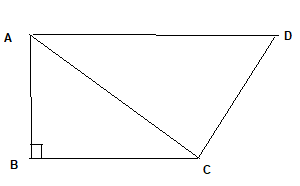It is given $\angle B = 90$
In right angle triangle ABC
$AC^2 = AB^2 + BC^2$ -(1)
Now it is given
$AD^2= AB^2 + BC^2 + CD^2$
From (1)
$AD^2 = AC^2 + CD^2$
By converse of Pythagoras theorem
$\angle ACD = 90$

Question 5.
ABC is a triangle in which AB = AC and D is a point on AC such that
$BC^2 =AC \times CD$ . Prove that BD=BC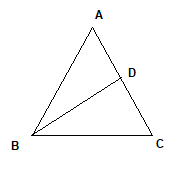Now given
$BC^2 =AC \times CD$
$\frac {BC}{AC} = \frac {CD}{BC}$

In $\Delta ABC$ and $\Delta BDC$
$\frac {BC}{AC} = \frac {CD}{BC}$
$\angle ACB = \angle BCD$
Hence
$\Delta ABC \sim \Delta BDC$
By CPCT
$\frac {BD}{BC} = \frac {AB}{AC}$
Now AB =AC
$\frac {BD}{BC}=1$
BD=BC

Question 6.
ABC is a right triangle right angled at C. Let BC = a, CA = b AB = c and let p be the length of perpendicular from C on AB, prove that
(i) $cp = ab$
(ii) $\frac {1}{p^2}= \frac {1}{a^2}+ \frac {1}{b^2}$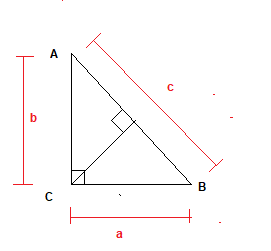Area of the Triangle ABC
$=\frac {1}{2} \times base \times height$
Now
Area of the Triangle ABC=$\frac {1}{2} \times a \times b= \frac {ab}{2}$
Now it can be also calculated as
Area of the Triangle ABC=$\frac {1}{2} \times c \times p= \frac {pc}{2}$
Since area is equal
$\frac {ab}{2}=\frac {pc}{2}$
$cp =ab$ -(1)

Now in right angle triangle ABC
$AC^2 = AB^2 + BC^2$
$c^2 = a^2 + b^2$
From (1)
$( \frac {ab}{p})^2 = a^2 + b^2$
$\frac {a^2b^2}{p^2} = a^2 + b^2$
Dividing by $a^2b^2$
$\frac {1}{p^2}= \frac {1}{a^2}+ \frac {1}{b^2}$

Question 7.
In the below figure PA, QB and RC are each perpendicular to AC.
Prove that
$\frac {1}{x} + \frac {1}{y} = \frac {1}{z}$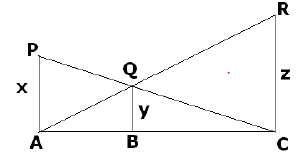This question is exactly similar to Question 2. Please check the solution

## Calculation Problems

Question 8.
if $\Delta ABC \sim \Delta DEF$ and their sides are of lengths (in cm) as marked along them, then find the lengths of the sides of each triangle.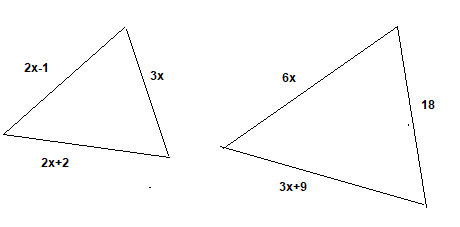Given
$\Delta ABC \sim \Delta DEF$
Therefore,
$\frac {AB}{DE} =\frac {BC}{EF}=\frac {CA}{FD}$
So,
$\frac {2x-1}{18}=\frac {2x+2}{3x+9}= \frac {3x}{6x}$
$\frac {2x-1}{18}=\frac {2x+2}{3x+9}$
or
$x = 5$
Therefore,
AB = 2 * 5 �1= 9, BC = 2 * 5 + 2 = 12,
CA = 3 * 5 = 15, DE = 18, EF = 3* 5 + 9 = 24 and FD = 6 * 5 = 30
Hence, AB = 9 cm, BC = 12 cm, CA = 15 cm
DE = 18 cm, EF = 24 cm and FD = 30 cm

Question 9.
Diagonals of a trapezium ABCD with AB||DC intersect each other at the point O. If AB = 2DC, find ratio of the areas of AOB and COD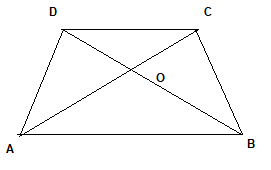In $\Delta AOB$ and $\Delta COD$, we have
$\angle AOB= \angle COD$ ( Vertically Opposite angles)
$\angle OCD= \angle OAB$ ( Alternate angles)
$\angle ODC=\angle OBA$ ( Alternate angles)
by AAA similarity
$\Delta AOB \sim \Delta COD$
Now Area of Similar Triangle is given by
$\frac {Area AOB}{Area COD} = \frac {AB^2}{CD^2}$
Now AB= 2CD
Hence
$\frac {Area AOB}{Area COD} = \frac {4}{1}$

## True and False

Question 10.
True and False statement
(b) All circles are similar.
(c ) All isosceles triangles are similar.
(d) All 30°, 60°, 90° triangles are similar.

a. False
b. True
c. False
d. True

## Fill in the blanks

Question 11.
(a) when two triangles are similar, then their corresponding altitudes are in the same _________.
(b) The ratio of the perimeters of two similar triangles is equal to the ratio of their corresponding _________.
(c) If in two triangles ABC and PQR,$\frac {AB}{QR}=\frac {BC}{PR}=\frac {CA}{PQ}$, then $\Delta PQR \sim$ _____.
(d) In two similar triangles, the corresponding angles are _________.

(a)ratio of the corresponding sides.
(b) sides
(c) $\Delta CAB$
(d) equal

## Summary

This Class 10 Maths Problems for Similar triangles with answers is prepared keeping in mind the latest syllabus of CBSE . This has been designed in a way to improve the academic performance of the students. If you find mistakes , please do provide the feedback on the mail.You can download in PDF form also using the below linksGo back to Class 10 Main Page using below links

### Practice Question

Question 1 What is $1 - \sqrt {3}$ ?
A) Non terminating repeating
B) Non terminating non repeating
C) Terminating
D) None of the above
Question 2 The volume of the largest right circular cone that can be cut out from a cube of edge 4.2 cm is?
A) 19.4 cm3
B) 12 cm3
C) 78.6 cm3
D) 58.2 cm3
Question 3 The sum of the first three terms of an AP is 33. If the product of the first and the third term exceeds the second term by 29, the AP is ?
A) 2 ,21,11
B) 1,10,19
C) -1 ,8,17
D) 2 ,11,20